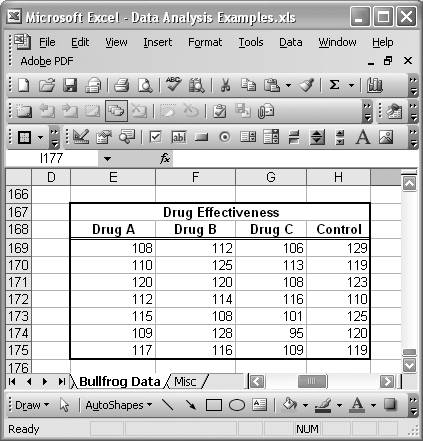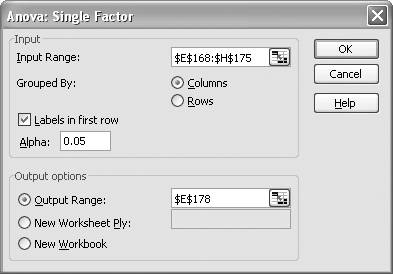# Conducting ANOVA

Problem

You'd like to conduct an analysis of variance (ANOVA).

Solution

The Analysis ToolPak offers three flavors of ANOVA: single factor, two-factor with replication, and two-factor without replication. Select Tools [Image_Link]/images/U2192.jpg border=0> Data Analysis from the main menu bar to access these tools.

Discussion

By way of example, consider the hypothetical drug effectiveness data shown in Figure 5-19.

The data values represent some measure of merit, or effectiveness, for drugs A, B, and C, along with a control group. The Anova: Single Factor tool allows you to perform an analysis of variance for two or more groups, so we'll use that tool to test the hypothesis that there's no difference between the means of these groups.

After selecting the Anova: Single Factor tool from the Data Analysis dialog box, you should see the Anova: Single Factor dialog box like the one shown in Figure 5-20.

In the Input Range field, type or select the cell range containing all the data under consideration. Your dataset must be in contiguous columns for this tool. If you include the column labels as I did in this example, then be sure to check the "Labels in first row" option. You can set the Alpha parameter to whatever you'd like. In this case I left it at 5%.

Finally, choose your output options and press OK. Here, I chose to generate the output in the same worksheet, starting in cell E178. The results of this analysis are shown in Figure 5-21.

These results show descriptive statistics for each group, along with standard ANOVA information. ANOVA results are displayed for between groups and within groups. SS represents the sum of squared deviations from the mean. df represents degrees of freedom. MS is the mean square value. F is the F-Ratio. And finally, F crit is the critical F-value based on the F-distribution. In this example, the P-value is less than the supplied Alpha value, and the obtained F-value is greater than the critical F-value. These results imply that we should reject the null hypothesis that there's no significant difference between the means of these groups and conclude that there's some statistically significant difference.

Figure 5-19. Drug effectivenessFigure 5-20. Anova: Single Factor dialog boxFigure 5-21. ANOVA resultsExcel Scientific and Engineering Cookbook (Cookbooks (OReilly))
ISBN: 0596008791
EAN: 2147483647
Year: N/A
Pages: 206
Authors: David M Bourg